Learn all Concepts of Chapter 8 Class 10 (with VIDEOS). Check - Trigonometry - Class 10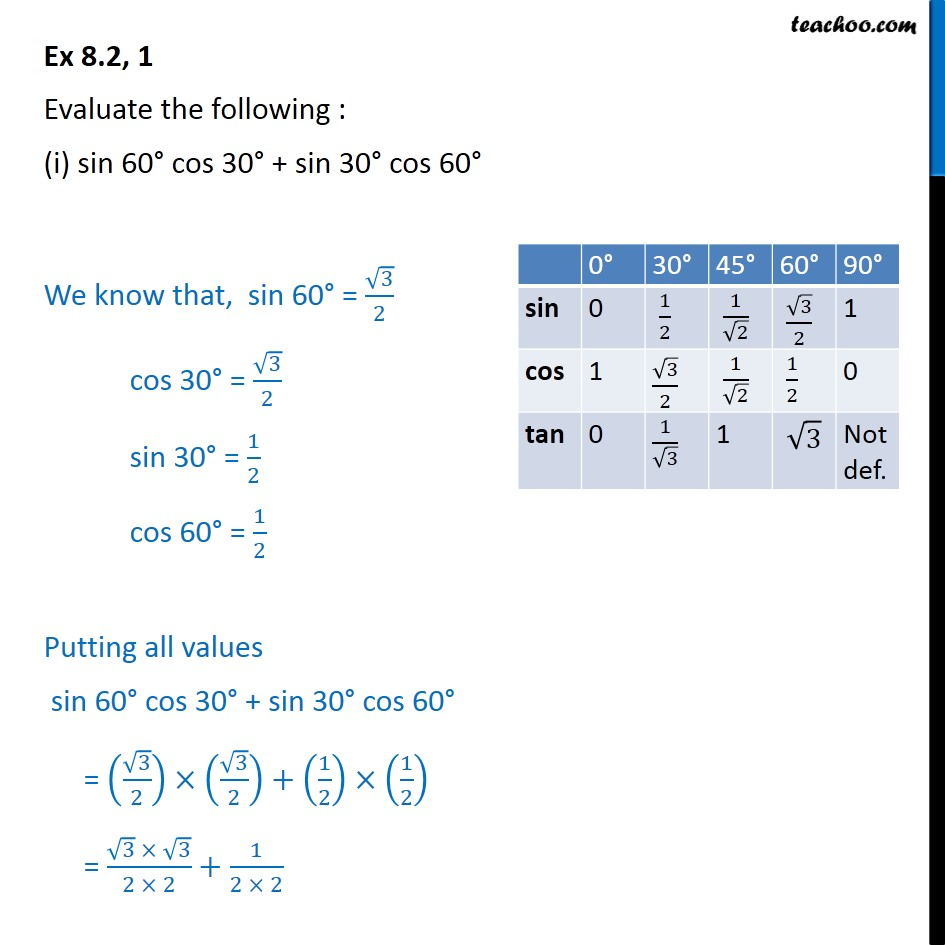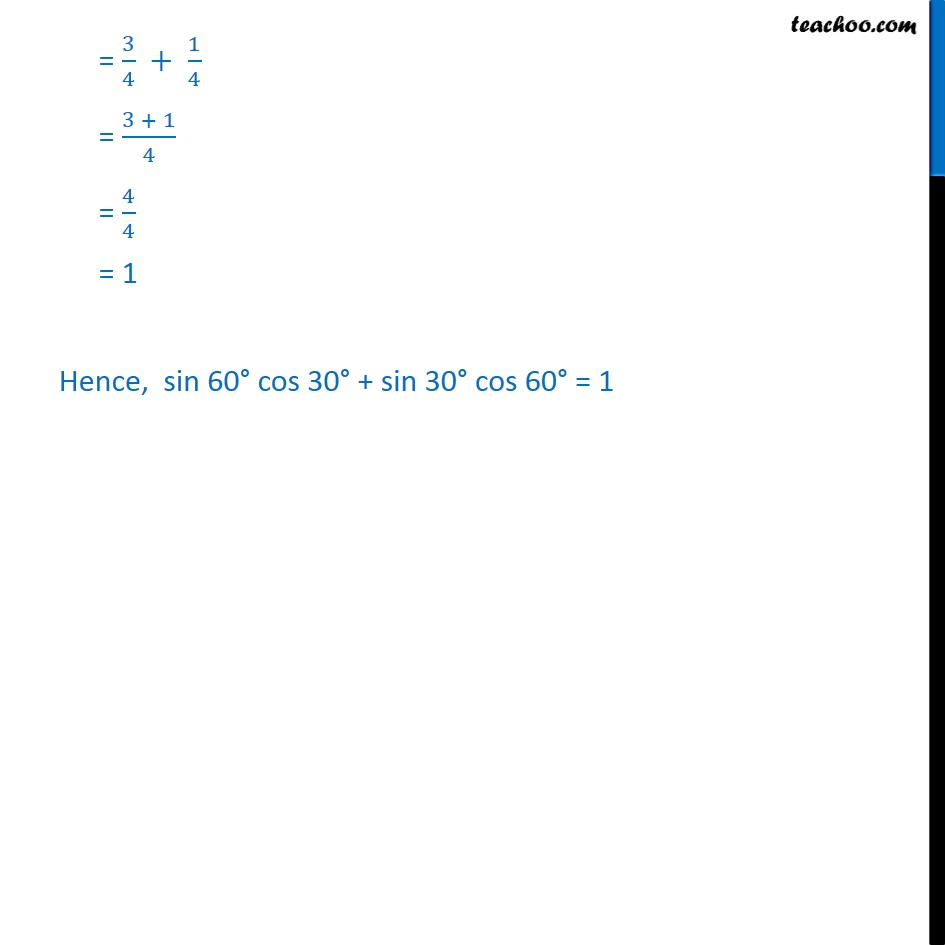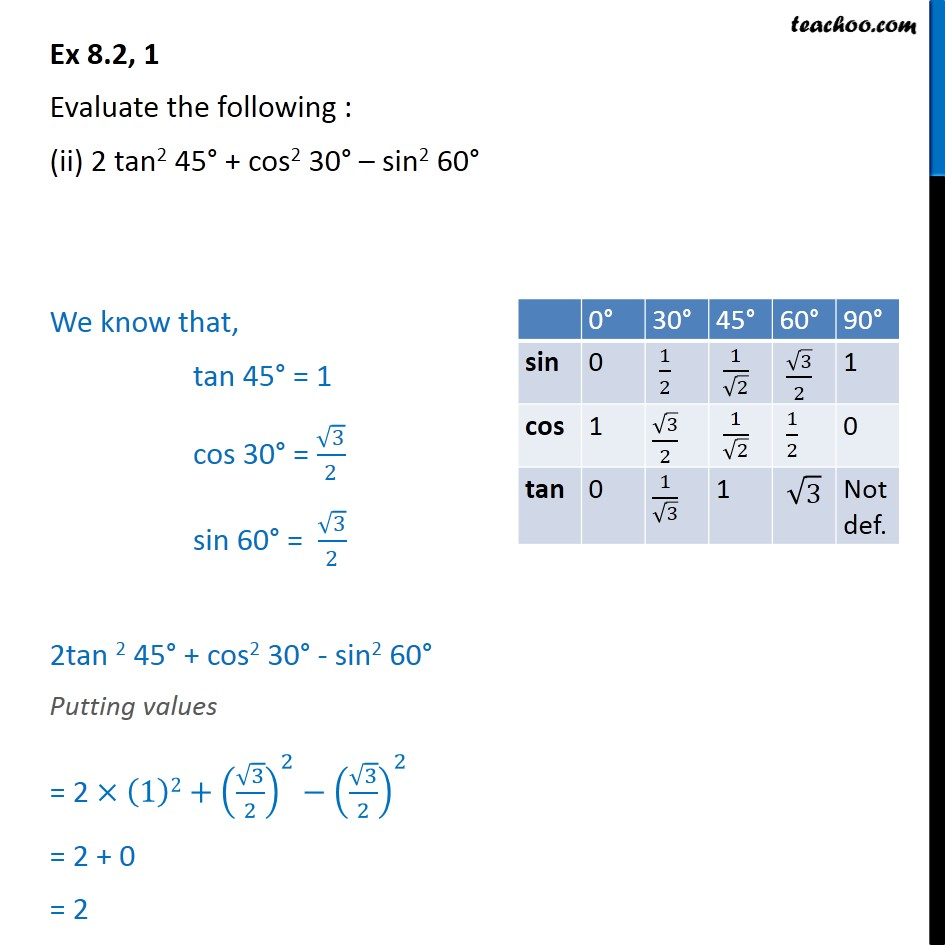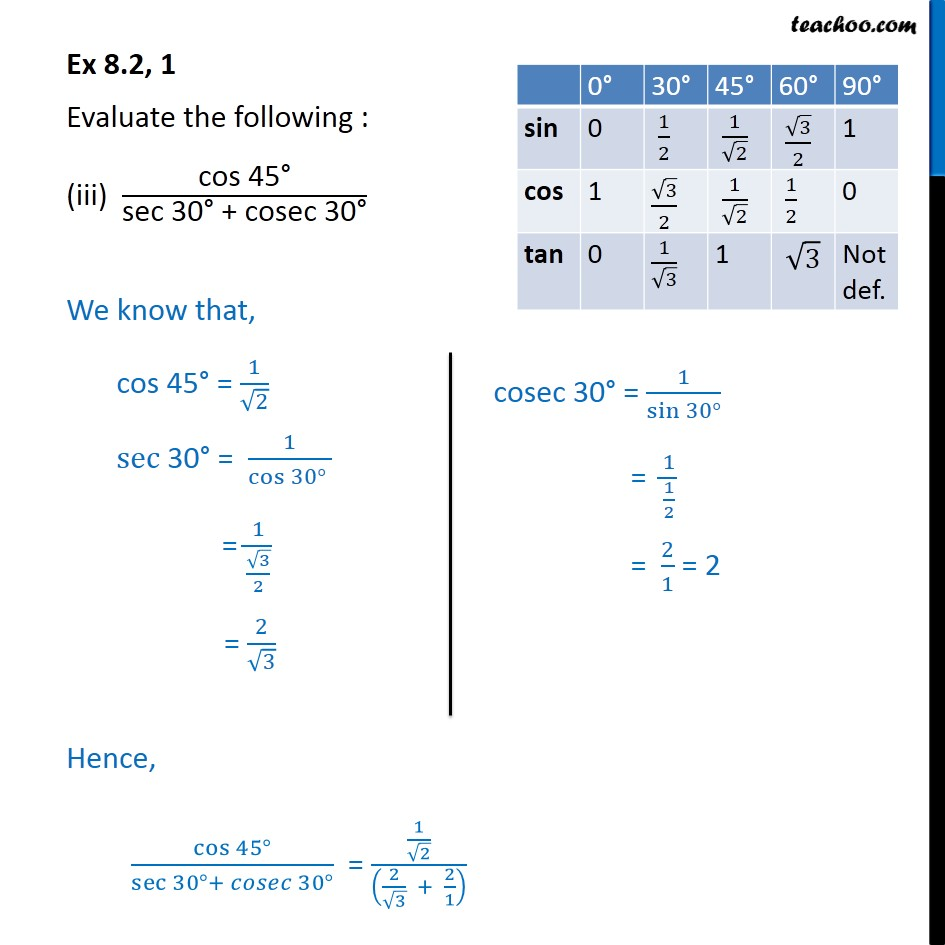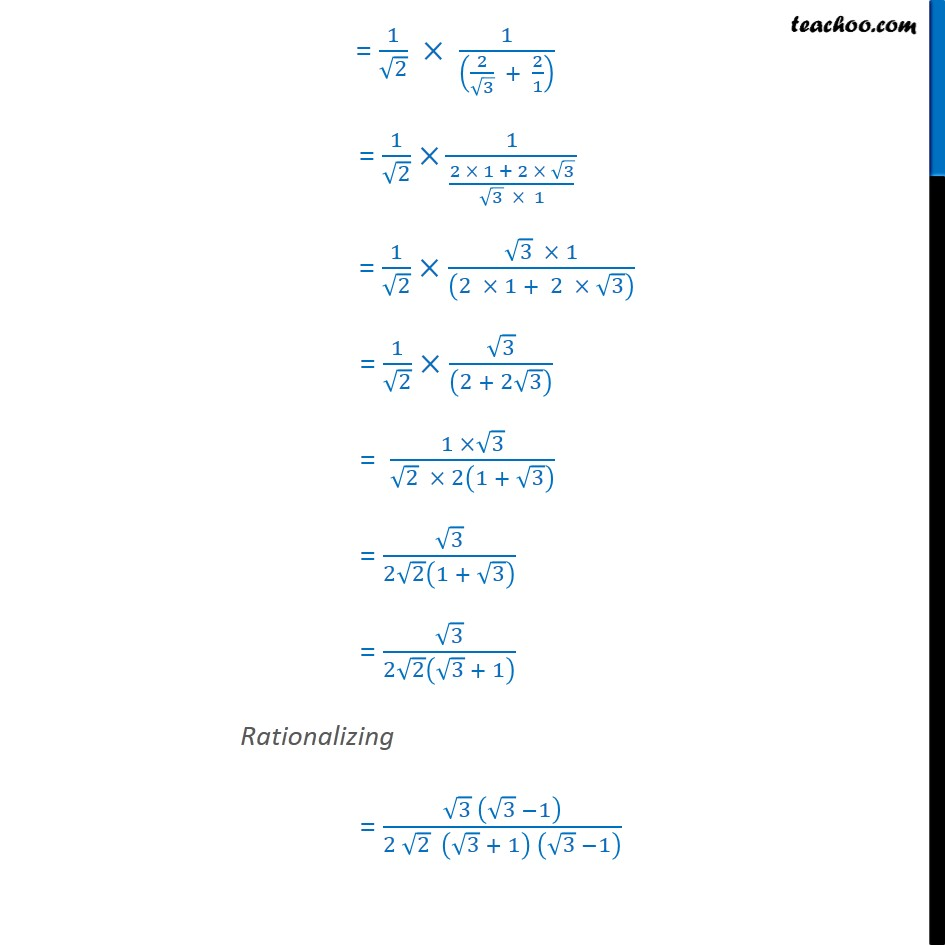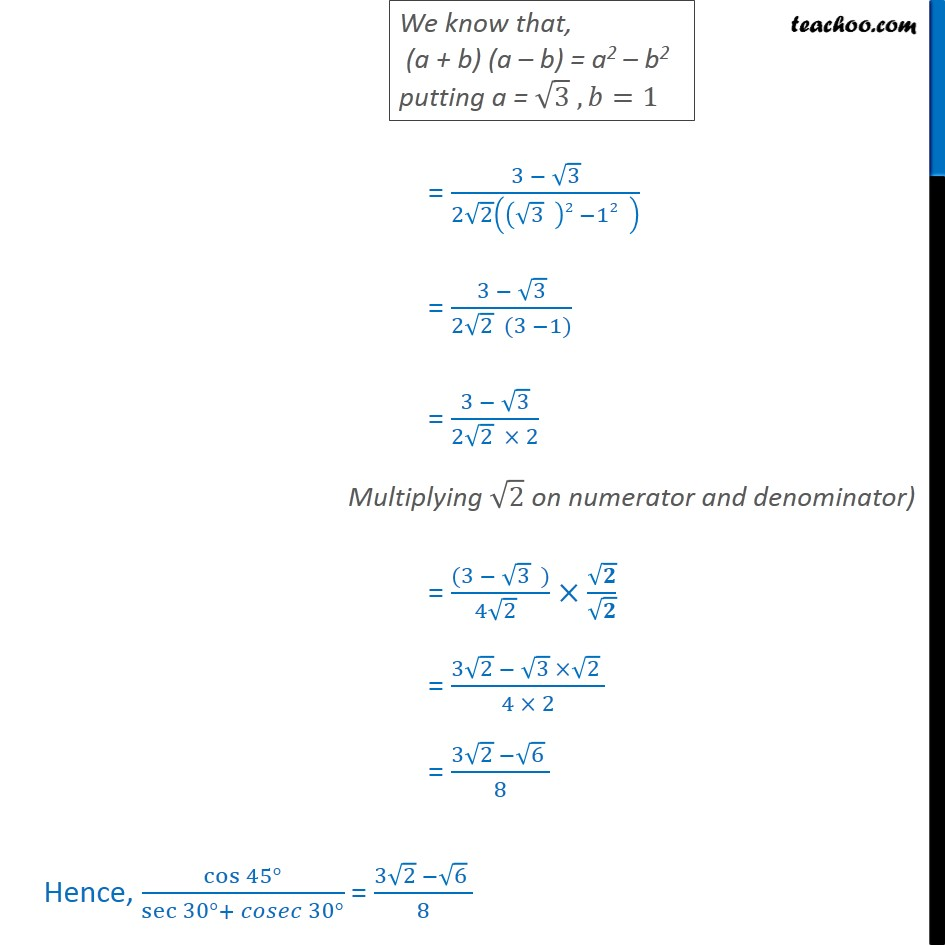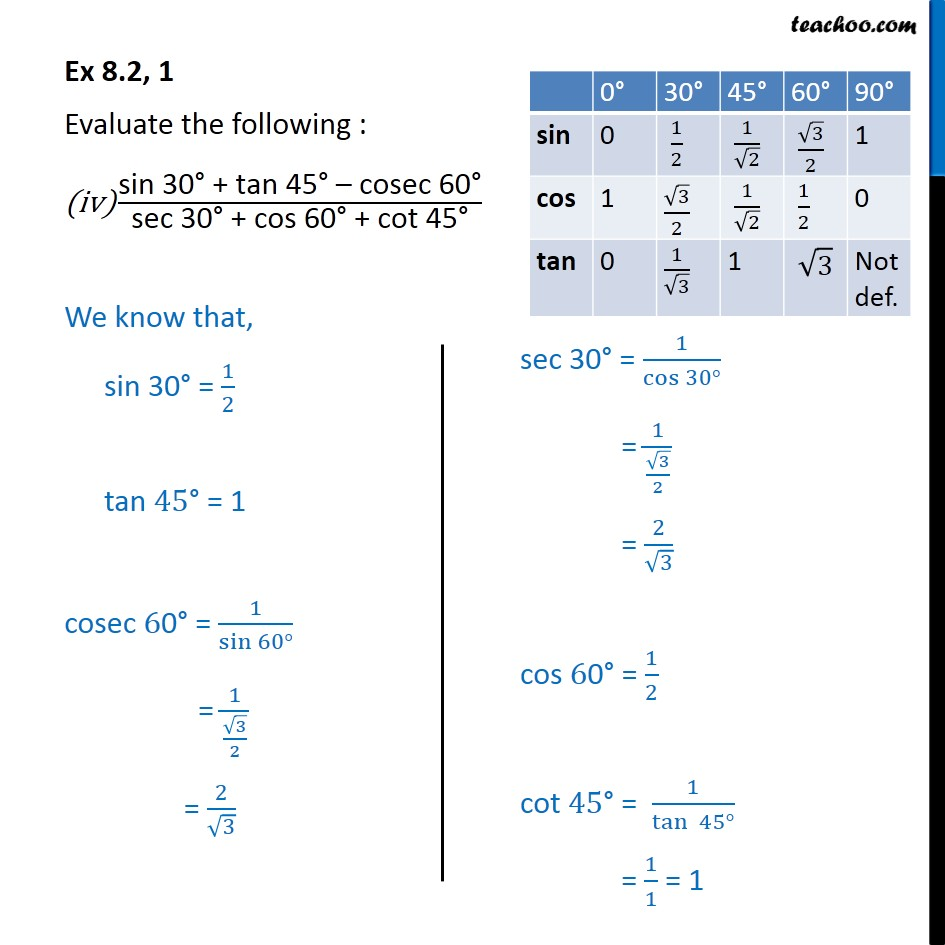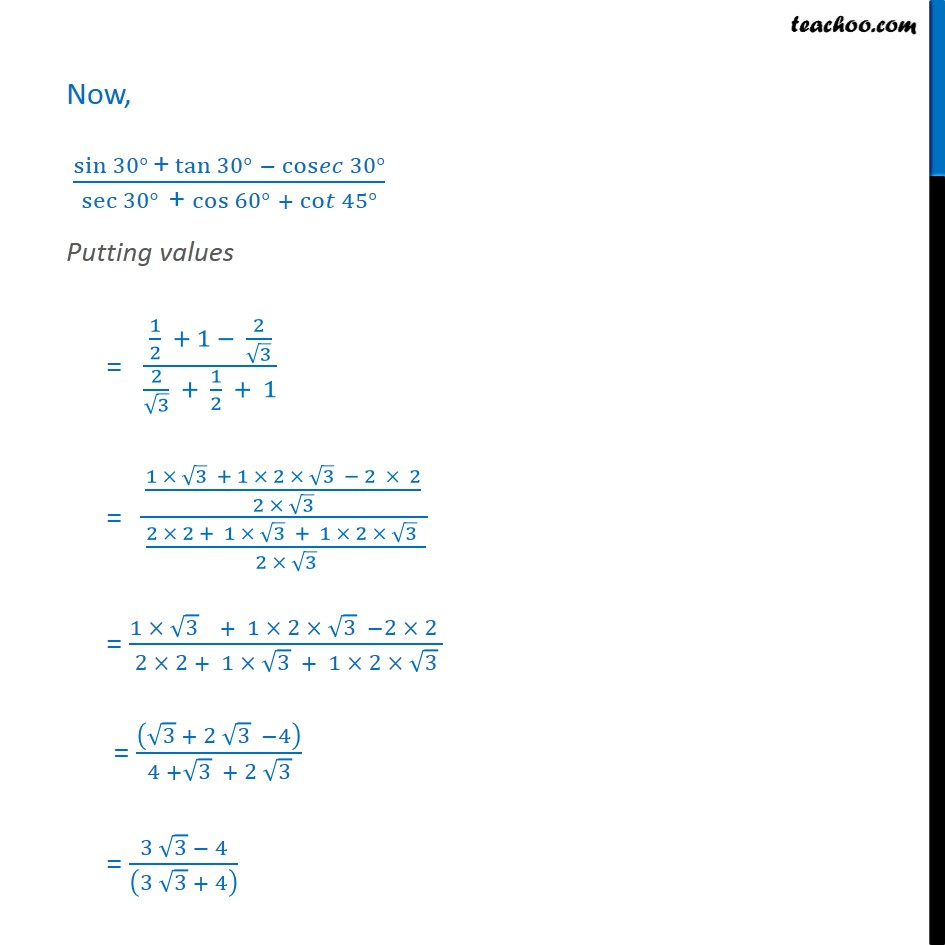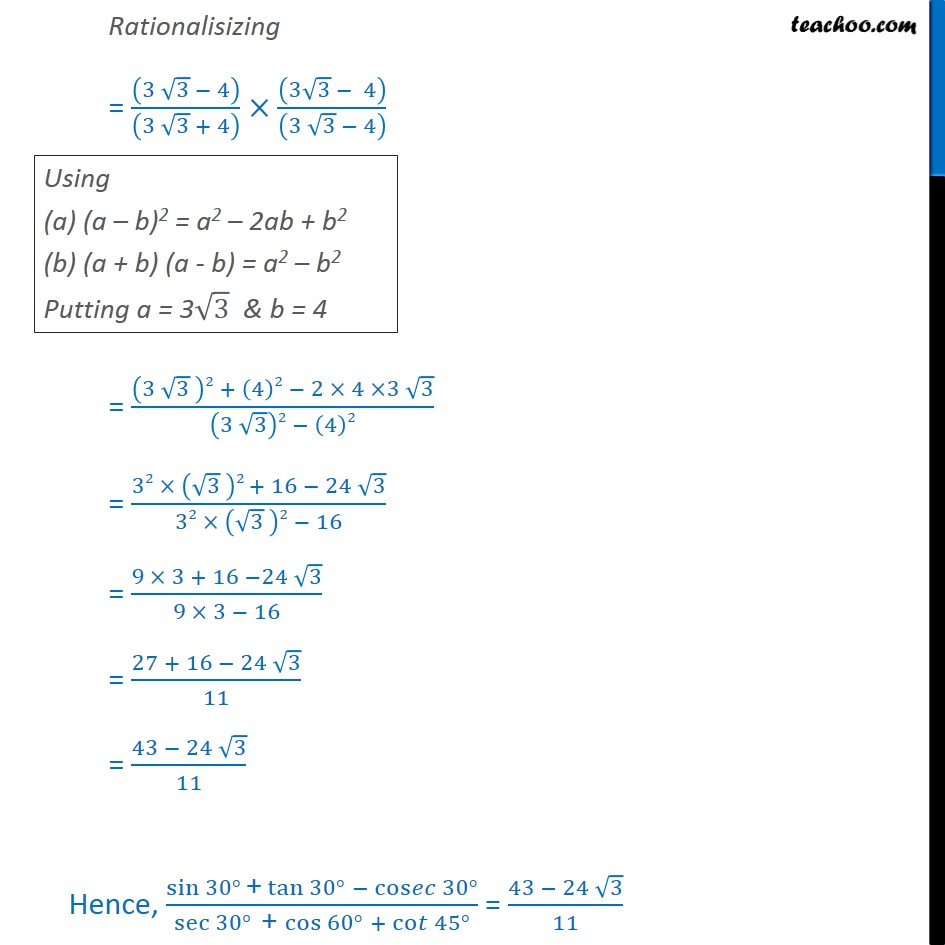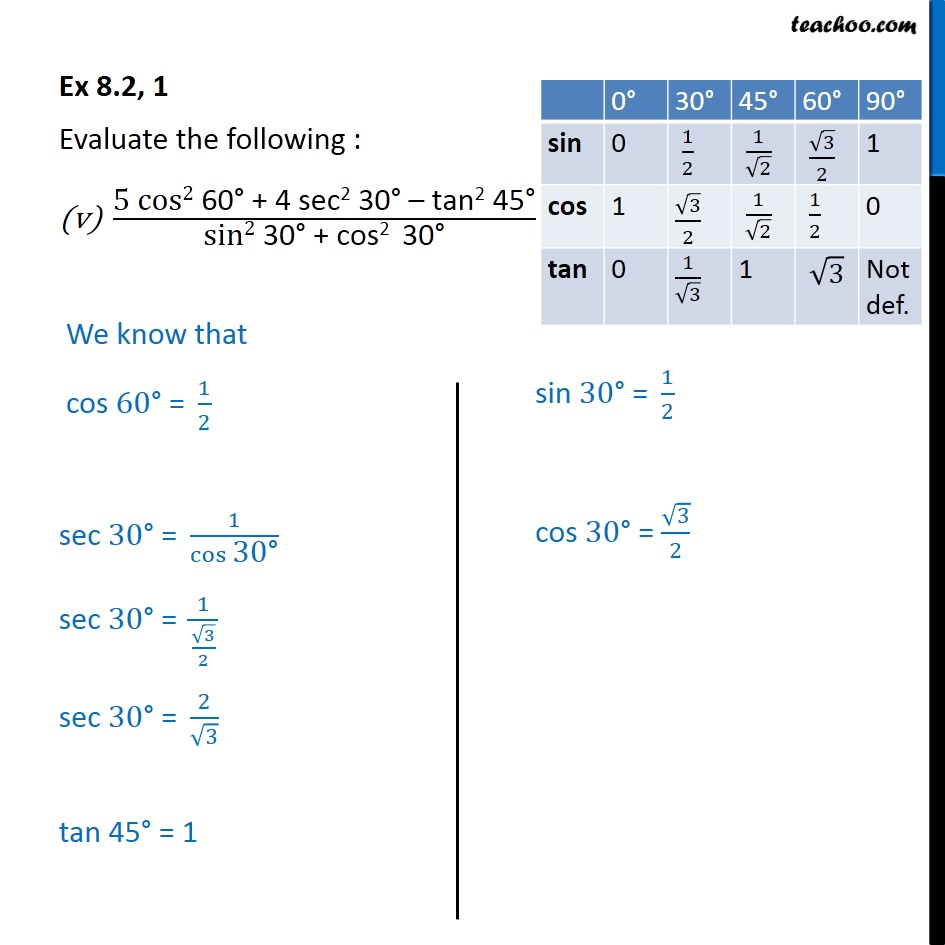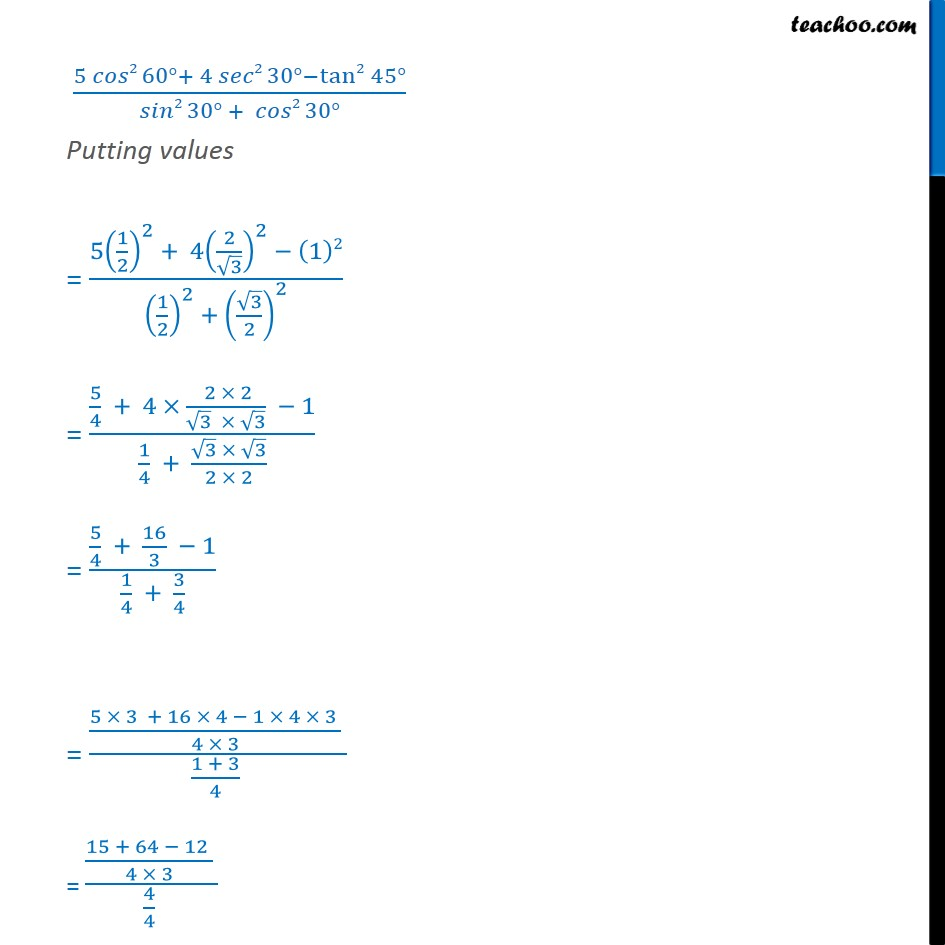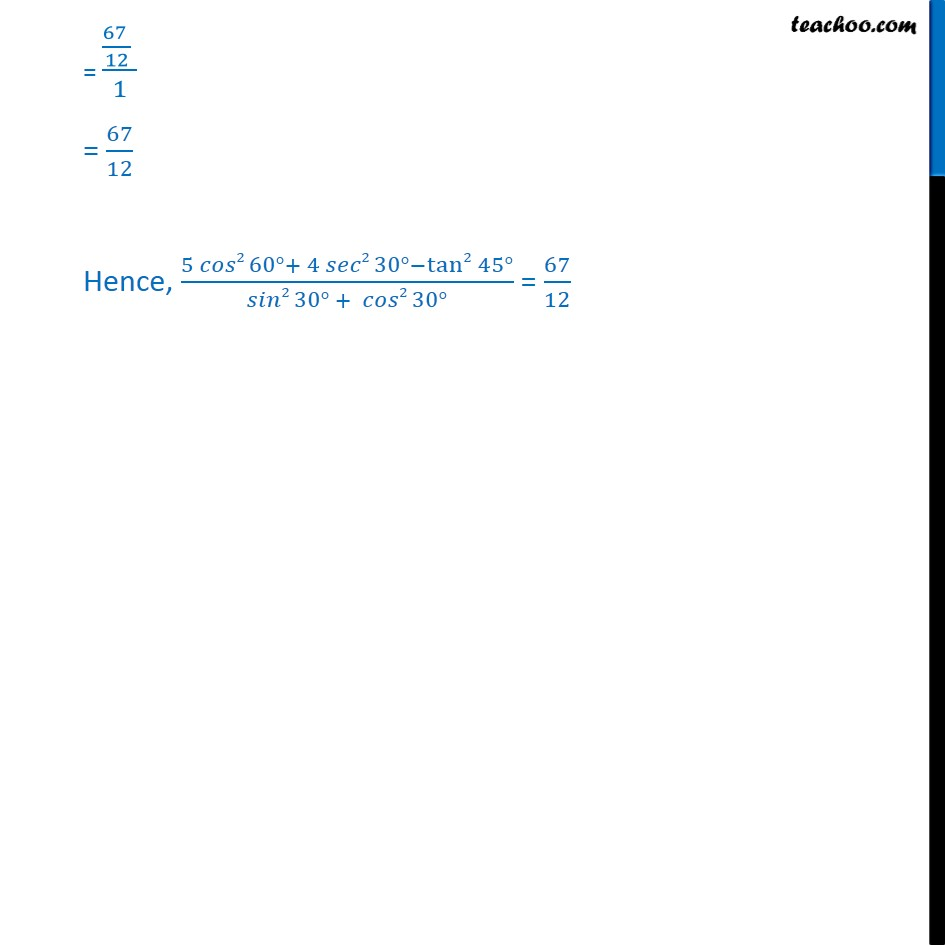1. Chapter 8 Class 10 Introduction to Trignometry
2. Serial order wise
3. Ex 8.2

Transcript

Ex 8.2, 1 Evaluate the following : (i) sin 60 cos 30 + sin 30 cos 60 We know that, sin 60 = 3/2 cos 30 = 3/2 sin 30 = 1/2 cos 60 = 1/2 Putting all values sin 60 cos 30 + sin 30 cos 60 = ( 3/2) ( 3/2)+(1/2) (1/2) = ( 3 3)/(2 2)+1/(2 2) = 3/4 + 1/4 = (3 + 1)/4 = 4/4 = 1 Hence, sin 60 cos 30 + sin 30 cos 60 = 1 Ex 8.2, 1 Evaluate the following : (ii) 2 tan2 45 + cos2 30 sin2 60 We know that, tan 45 = 1 cos 30 = 3/2 sin 60 = 3/2 2tan 2 45 + cos2 30 - sin2 60 Putting values = 2 (1)2+( 3/2)^2 ( 3/2)^2 = 2 + 0 = 2 Ex 8.2, 1 Evaluate the following : (iii) "cos 45 " /"sec 30 + cosec 30 " We know that, "cos 45 " = 1/ 2 "sec 30 " = 1/(cos 30 ) = 1/( 3/2) = 2/ 3 Hence, cos 45 /(sec 30 + 30 ) = (1/ 2)/((2/ 3 + 2/1) ) = 1/ 2 1/((2/ 3 + 2/1) ) = 1/ 2 1/((2 1 + 2 3)/( 3 1)) = 1/ 2 ( 3 1)/((2 1 + 2 3) ) = 1/ 2 3/((2 + 2 3) ) = (1 3)/( 2 2(1 + 3) ) = 3/(2 2 (1 + 3) ) = 3/(2 2 ( 3 + 1) ) Rationalizing = ( 3 ( 3 1))/(2 2 ( 3 + 1) ( 3 1) ) = (3 3)/(2 2 (( 3 )2 12 ) ) = (3 3)/(2 2 (3 1)) = (3 3)/(2 2 2) Multiplying 2 on numerator and denominator) = ((3 3 ))/(4 2 ) / = (3 2 3 2 )/(4 2) = (3 2 6 )/8 Ex 8.2, 1 Evaluate the following : "sin 30 + tan 45 cosec 60 " /"sec 30 + cos 60 + cot 45 " We know that, sin "30 " = 1/2 tan "45 " = 1 cosec 6"0 " = 1/sin 60 = 1/( 3/2) = 2/ 3 Now, (sin 30 "+" tan 30 " " cos 30 )/(sec 30 "+ " cos 60 " " + co 45 ) Putting values = (1/2 + 1 2/ 3)/(2/ 3 + 1/2 + 1) = ((1 3 + 1 2 3 2 2)/(2 3) )/((2 2 + 1 3 + 1 2 3 )/(2 3)) = (1 3 + 1 2 3 2 2 )/(2 2 + 1 3 + 1 2 3) = (( 3 + 2 3 4))/(4 + 3 + 2 3) = (3 3 4)/((3 3 + 4) ) Rationalisizing = ((3 3 4))/((3 3 + 4) ) ((3 3 4))/((3 3 4) ) = ((3 3 )2 + (4)2 2 4 3 3)/((3 3)2 (4)2) = (32 ( 3 )2 + 16 24 3)/(32 ( 3 )2 16) = (9 3 + 16 24 3)/(9 3 16) = (27 + 16 24 3)/11 = (43 24 3)/11 Hence, (sin 30 "+" tan 30 " " cos 30 )/(sec 30 "+ " cos 60 " " + co 45 ) = (43 24 3)/11 Ex 8.2, 1 Evaluate the following : "5 cos2 60 + 4 sec2 30 tan2 45 " /"sin2 30 + cos2 30 " We know that cos "60 = " 1/2 sec "30 = " 1/cos "30 " sec "30 = " 1/( 3/2) sec "30 = " 2/ 3 tan "45 " = 1 (5 2 60 + 4 2 30 tan2 45 )/( 2 30 + 2 30 ) Putting values = (5(1/2)^2 + 4(2/ 3)^2 (1)2)/((1/2)^2 + ( 3/2)^2 ) = (5/4 + 4 ( 2 2)/( 3 3) 1)/(1/4 + ( 3 3)/(2 2)) = (5/4 + 16/3 1)/(1/4 + 3/4) = ((5 3 + 16 4 1 4 3 )/(4 3) )/((1 + 3)/4 ) = ((15 + 64 12 )/(4 3) )/(4/4 ) = ((67 )/12 )/1 = 67/12 Hence, (5 2 60 + 4 2 30 tan2 45 )/( 2 30 + 2 30 ) = 67/12

Ex 8.2